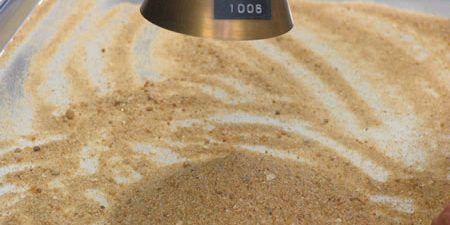# Determination of Absorption of Fine Aggregate

Introduction:

The absorption of fine aggregate is an essential parameter to consider when designing concrete mixtures. It is defined as the percentage of water absorbed by the fine aggregate at a saturated surface-dry (SSD) condition. In this article, we will discuss the ASTM standard method for the determination of the absorption of fine aggregate.

## Materials:

• Oven
• Balance
• Saturated surface-dry (SSD) fine aggregate
• Water
• Container

## Procedure:

1. Weigh the container and record its mass as A.
2. Fill the container with water up to about one-third of its capacity.
3. Add a known mass of SSD fine aggregate to the container until the water level rises to approximately two-thirds of the container’s capacity. (Learn the Procedure for Attaining Saturated Surface Dry Conditions of Fine Aggregate)
4. Mix the fine aggregate and water in the container thoroughly.
5. Allow the mixture to stand for 24 ± 4 hours at a temperature of 22 ± 2°C.
6. After 24 ± 4 hours, remove the excess water from the container’s surface with a towel or blotting paper.
7. Weigh the container with the remaining fine aggregate and record its mass as S.
8. Dry the fine aggregate in the oven to a constant mass at a temperature of 110 ± 5°C for at least 24 hours.
9. Weigh the dried fine aggregate and record its mass as D.

## Calculations:

1. Calculate the mass of water absorbed by the fine aggregate using the formula: Water Absorbed = S – D
2. Calculate the absorption of the fine aggregate using the formula: Absorption = (Water Absorbed / D) x 100

## Results:

Report the absorption of the fine aggregate as a percentage to the nearest 0.1%.

## Conclusion:

The absorption of fine aggregate is an important factor to consider when designing concrete mixtures. By following the ASTM standard method for the determination of absorption, accurate and reliable results can be obtained.